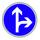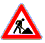# Previously teaching

Math 223M, Hardy fields (Winter Quarter 2020)
Math 220A, Mathematical Logic and Set Theory (Fall Quarter 2019)
Math 223M, Topics in Model Theory (Fall Quarter 2018)
Math 131A, Real Analysis, lecture 3 (Spring Quarter 2018)
Math 131A, Real Analysis, lecture 4 (Spring Quarter 2018)
Math 223M, Topics in Model Theory (Fall Quarter 2017)
Math 110A, Algebra (Fall Quarter 2017)
Math 223M, Topics in Model Theory (Spring Quarter 2017)
Math 31A, Differential and Integral Calculus (Winter Quarter 2017)
Math 220A, Mathematical Logic and Set Theory (Fall Quarter 2016)
Math 31B, Integration and Infinite Series (Spring Quarter 2016)
Math 220B, Mathematical Logic and Set Theory (Winter Quarter 2016)
Math 31A, Differential and Integral Calculus (Fall Quarter 2015)
Math 220A, Mathematical Logic and Set Theory (Fall Quarter 2015)
Math 131A, Real Analysis (Spring Quarter 2015)
Math 223M, Model Theory (Spring Quarter 2015)
Math 31A, Differential and Integral Calculus (Winter Quarter 2015)
Math 285D, Surreal Numbers (Fall Quarter 2014)
Math 131A, Real Analysis (Fall Quarter 2013)
Math 31B, Integration and Infinite Series (Fall Quarter 2013)
Math 31B,  Integration and Infinite Series (Spring Quarter 2013)
Math 220B, Mathematical Logic and Set Theory (Winter Quarter 2013)
Math 110A, Algebra (Fall Quarter 2012)
Math 220A, Mathematical Logic and Set Theory (Fall Quarter 2012)
Math 131A,  Real Analysis (Spring Quarter 2012)
Math 290C (jointly with Raphael Rouquier), Motivic Integration (Spring Quarter 2012)
Math 223M,  Model Theory (Winter Quarter 2012)
Math 31A, Differential and Integral Calculus (Fall Quarter 2011)
Math 31B,  Integration and Infinite Series (Spring Quarter 2011)
Math 31A,  Differential and Integral Calculus (Winter Quarter 2011)
Math 223M,  Topics in Model Theory: O-Minimality and Diophantine Geometry (Winter Quarter 2011)
Math 223M,  Model Theory (Spring Quarter 2010)
Math 31A,  Differential and Integral Calculus (Fall Quarter 2009)
Math 223M,  Model Theory (Spring Quarter 2009)
Math 31B,  Integration and Infinite Series (Fall Quarter 2008)
Math 114L,  Mathematical Logic (Spring Quarter 2008)
Math 285D, An Introduction to O-Minimality (Winter Quarter 2008)
Math 191, Algorithms for Elementary Algebraic Geometry (Fall Quarter 2007)

At UIC:

Math 180, Calculus I (Fall 2006)
Math 435, Foundations of Number Theory (Fall 2006)
Math 503, Metamathematics II (Spring 2006)
Math 181, Calculus II (Fall 2005)
Math 512, Advanced Topics in Logic: Model Theory of Valued Fields (Fall 2005)
Math 430, Formal Logic (Fall 2004)
Math 512, Advanced Topics in Logic: Model Theory of Real Exponentiation (Fall 2004)
Math 506, Model Theory (Spring 2004)
Math 180, Calculus I (Fall 2003)Last modified March 24, 2020.